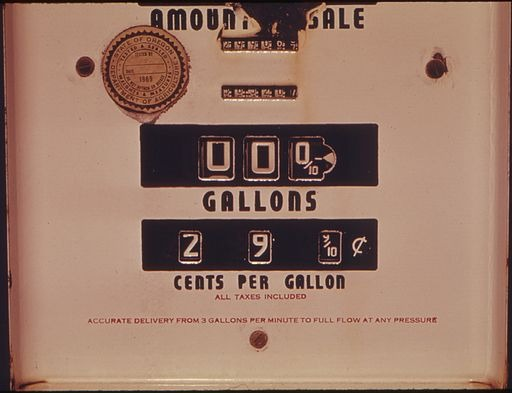# Defining Rate Discussion# Using Mathematical Definitions

Attending to precision is an important mathematical practice. One of the most important parts of attending to precision is developing and using clear definitions. In this lesson, you will write a definition of rate and then apply the definition to see if it is precise enough to be useful.

Think about and use this mathematical practice of attending to precision in this class, in other math classes that you take, and as you use mathematics in your life.

• How is the conversation an example of attending to precision?